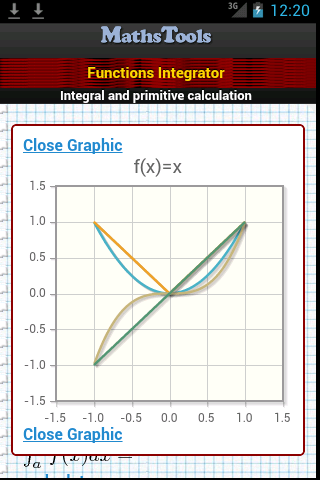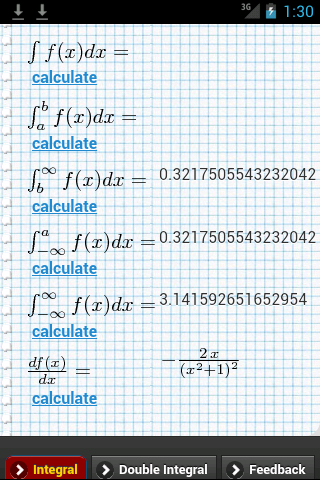# Functions Integrator Mobile for Android devices

### Basics Concepts and Principles

Functions Integrator is an online tool that allows calculate indefinite, definite, semi-infinite or infinite integrals

Functions in the form

f: R → R
(x) → y=f(x)

Functions Integrator also solve Differential Ordinary Equations. Try it!Functions Integrator makes graphics of functions f(x) in interval [a, b] Functions Integrator calculates indefinite integrals or primitive functions.Functions Integrator calculates integrals in infinite or semi-infinite intervals. Functions Integrator calculate double integral for f(x,.y) functions

Try to solve your Differential Equations up to order 2 with Functions Integrator.

## Was useful? want add anything?

Post here

### Post from other users

Post here